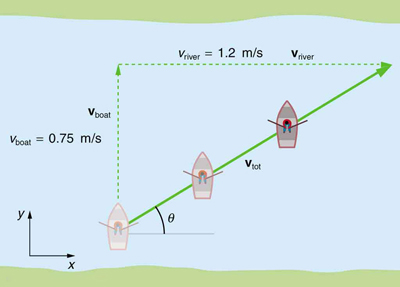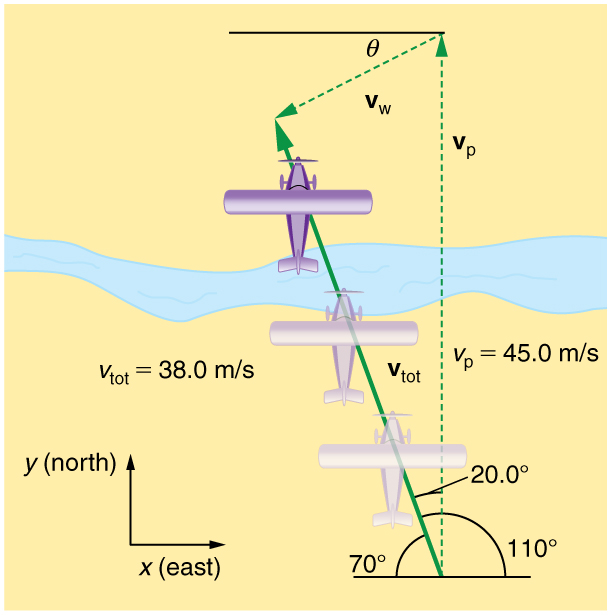# 3.5 Addition of velocities  (Page 2/12)

 Page 2 / 12

## Take-home experiment: relative velocity of a boat

Fill a bathtub half-full of water. Take a toy boat or some other object that floats in water. Unplug the drain so water starts to drain. Try pushing the boat from one side of the tub to the other and perpendicular to the flow of water. Which way do you need to push the boat so that it ends up immediately opposite? Compare the directions of the flow of water, heading of the boat, and actual velocity of the boat.

## Adding velocities: a boat on a riverA boat attempts to travel straight across a river at a speed 0.75 m/s. The current in the river, however, flows at a speed of 1.20 m/s to the right. What is the total displacement of the boat relative to the shore?

Refer to [link] , which shows a boat trying to go straight across the river. Let us calculate the magnitude and direction of the boat’s velocity relative to an observer on the shore, ${\mathbf{\text{v}}}_{\text{tot}}$ . The velocity of the boat, ${\mathbf{\text{v}}}_{\text{boat}}$ , is 0.75 m/s in the $y$ -direction relative to the river and the velocity of the river, ${\mathbf{\text{v}}}_{\text{river}}$ , is 1.20 m/s to the right.

Strategy

We start by choosing a coordinate system with its $x$ -axis parallel to the velocity of the river, as shown in [link] . Because the boat is directed straight toward the other shore, its velocity relative to the water is parallel to the $y$ -axis and perpendicular to the velocity of the river. Thus, we can add the two velocities by using the equations ${v}_{\text{tot}}=\sqrt{{v}_{x}^{2}+{v}_{y}^{2}}$ and $\theta ={\text{tan}}^{-1}\left({v}_{y}/{v}_{x}\right)$ directly.

Solution

The magnitude of the total velocity is

${v}_{\text{tot}}=\sqrt{{v}_{x}^{2}+{v}_{y}^{2}}\text{,}$

where

${v}_{x}={v}_{\text{river}}=1\text{.}\text{20 m/s}$

and

${v}_{y}={v}_{\text{boat}}=0\text{.}\text{750 m/s.}$

Thus,

${v}_{\text{tot}}=\sqrt{\left(1\text{.}\text{20 m/s}{\right)}^{2}+\left(0\text{.}\text{750 m/s}{\right)}^{2}}$

yielding

${v}_{\text{tot}}=1\text{.}\text{42 m/s.}$

The direction of the total velocity $\theta$ is given by:

$\theta ={\text{tan}}^{-1}\left({v}_{y}/{v}_{x}\right)={\text{tan}}^{-1}\left(0\text{.}\text{750}/1\text{.}\text{20}\right)\text{.}$

This equation gives

$\theta =\text{32}\text{.}0º\text{.}$

Discussion

Both the magnitude $v$ and the direction $\theta$ of the total velocity are consistent with [link] . Note that because the velocity of the river is large compared with the velocity of the boat, it is swept rapidly downstream. This result is evidenced by the small angle (only $32.0º$ ) the total velocity has relative to the riverbank.

## Calculating velocity: wind velocity causes an airplane to drift

Calculate the wind velocity for the situation shown in [link] . The plane is known to be moving at 45.0 m/s due north relative to the air mass, while its velocity relative to the ground (its total velocity) is 38.0 m/s in a direction $\text{20}\text{.0º}$ west of north.An airplane is known to be heading north at 45.0 m/s, though its velocity relative to the ground is 38.0 m/s at an angle west of north. What is the speed and direction of the wind?

Strategy

In this problem, somewhat different from the previous example, we know the total velocity ${\mathbf{\text{v}}}_{\text{tot}}$ and that it is the sum of two other velocities, ${\mathbf{\text{v}}}_{\text{w}}$ (the wind) and ${\mathbf{\text{v}}}_{\text{p}}$ (the plane relative to the air mass). The quantity ${\mathbf{\text{v}}}_{\text{p}}$ is known, and we are asked to find ${\mathbf{\text{v}}}_{\text{w}}$ . None of the velocities are perpendicular, but it is possible to find their components along a common set of perpendicular axes. If we can find the components of ${\mathbf{\text{v}}}_{\text{w}}$ , then we can combine them to solve for its magnitude and direction. As shown in [link] , we choose a coordinate system with its x -axis due east and its y -axis due north (parallel to ${\mathbf{\text{v}}}_{\text{p}}$ ). (You may wish to look back at the discussion of the addition of vectors using perpendicular components in Vector Addition and Subtraction: Analytical Methods .)

tree physical properties of heat
tree is a type of organism that grows very tall and have a wood trunk and branches with leaves... how is that related to heat? what did you smoke man?
what are the uses of dimensional analysis
Dimensional Analysis. The study of relationships between physical quantities with the help of their dimensions and units of measurements is called dimensional analysis. We use dimensional analysis in order to convert a unit from one form to another.
Emmanuel
meaning of OE and making of the subscript nc
Negash
kinetic functional force
what is a principal wave?
A wave the movement of particles on rest position transferring energy from one place to another
Gabche
not wave. i need to know principal wave or waves.
Haider
principle wave is a superposition of wave when two or more waves meet at a point , whose amplitude is the algebraic sum of the amplitude of the waves
kindly define principal wave not principle wave (principle of super position) if u can understand my question
Haider
what is a model?
hi
Muhanned
why are electros emitted only when the frequency of the incident radiation is greater than a certain value
b/c u have to know that for emission of electron need specific amount of energy which are gain by electron for emission . if incident rays have that amount of energy electron can be emitted, otherwise no way.
Nazir
Nazir
what is ohm's law
states that electric current in a given metallic conductor is directly proportional to the potential difference applied between its end, provided that the temperature of the conductor and other physical factors such as length and cross-sectional area remains constant. mathematically V=IR
ANIEFIOK
hi
Gundala
A body travelling at a velocity of 30ms^-1 in a straight line is brought to rest by application of brakes. if it covers a distance of 100m during this period, find the retardation.
just use v^2-u^2=2as
Gundala
how often does electrolyte emits?
alhassan
just use +€^3.7°√π%-4¢•∆¥%
v^2-u^2=2as v=0,u=30,s=100 -30^2=2a*100 -900=200a a=-900/200 a=-4.5m/s^2
akinyemi
what's acceleration
The change in position of an object with respect to time
Mfizi
Acceleration is velocity all over time
Pamilerin
hi
Stephen
It's not It's the change of velocity relative to time
Laura
Velocity is the change of position relative to time
Laura
acceleration it is the rate of change in velocity with time
Stephen
acceleration is change in velocity per rate of time
Noara
what is ohm's law
Stephen
Ohm's law is related to resistance by which volatge is the multiplication of current and resistance ( U=RI)
Laura
acceleration is the rate of change. of displacement with time.
the rate of change of velocity is called acceleration
Asma
how i don understand
how do I access the Multiple Choice Questions? the button never works and the essay one doesn't either
How do you determine the magnitude of force
mass × acceleration OR Work done ÷ distance
Seema
Which eye defect is corrected by a lens having different curvatures in two perpendicular directions?
acute astigmatism?ByByBy Kevin AmaratungaBy Anindyo MukhopadhyayBy OpenStaxBy OpenStaxBy Miranda ReisingBy Lakeima RobertsBy Tamsin KnoxBy Yasser IbrahimBy OpenStaxBy Steve Gibbs